# Overall of IBEW Aptitude Tests: Process, Guide & Examples

IBEW (International Brotherhood of Electrical Workers) is a desirable workplace for anyone who is vying for a career in the electricity industry. To pick out the best-suited candidates from a vast number of applications every year, the company uses IBEW Aptitude Tests, a comprehensive tool to measure pre-hires competencies.

## What are IBEW aptitude tests?

IBEW aptitude tests are assessment tools that IBEW (International Brotherhood of Electrical Workers) uses to screen candidates for electrician apprenticeships. The tests are divided into 2 main types:

• Math: includes algebra and number series questions, assessing candidates’ problem-solving and math skills.
• Reading: requires you to infer information from a passage, measuring your ability to process written data.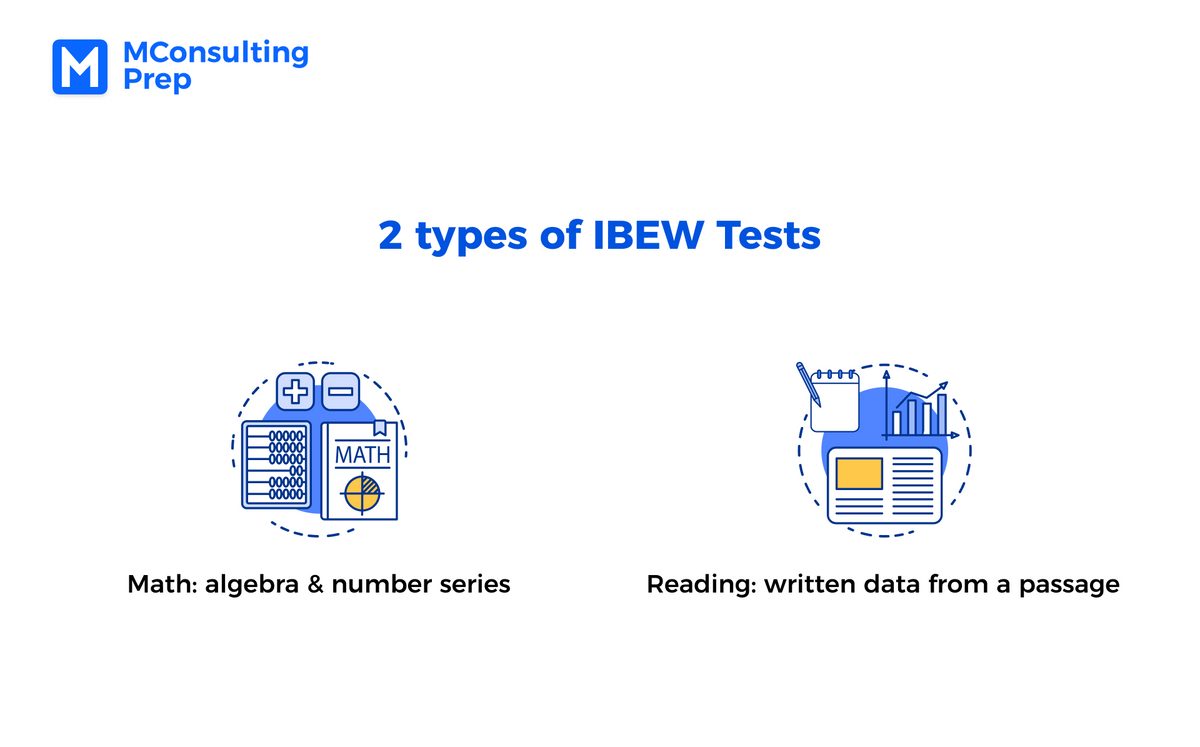What do IBEW aptitude tests assess?

IBEW aptitude tests aim to test two domains of candidates’ cognitive ability: numeracy skills and reading comprehension. Besides aptitude tests, IBEW assessments can also include mechanical questions to assess your technical knowledge.

Which positions are IBEW aptitude tests for?

IBEW aptitude tests are designed for anyone starting their career as a licensed electrician by applying for an apprenticeship in the organization. Several trades require IBEW aptitude tests are:

• Inside Wireman
• Construction Electrician
• Electrical Trainee
• Sound & Communication Installers

Are IBEW aptitude tests hard?

IBEW aptitude tests are considered tough challenges for many candidates, especially the math section. Unlike aptitude tests from other hiring companies, math tests at IBEW comprise algebra and functions, which require a solid foundation in math.

What is the passing score for IBEW aptitude tests?

The qualifying score for most apprenticeships at IBEW is 4/9. However, you should achieve above average as job vacancies at IBEW are limited, and the company will pick out candidates with the best performance to join the team.

## When do IBEW aptitude tests take place?

IBEW Aptitude Tests are in the second stage of the IBEW hiring process. If you aim to join the company, it is crucial to prepare carefully for all 3 stages of the selection: Application ⇒ Aptitude Tests ⇒ Interview. Here is a brief description of the recruitment at IBEW: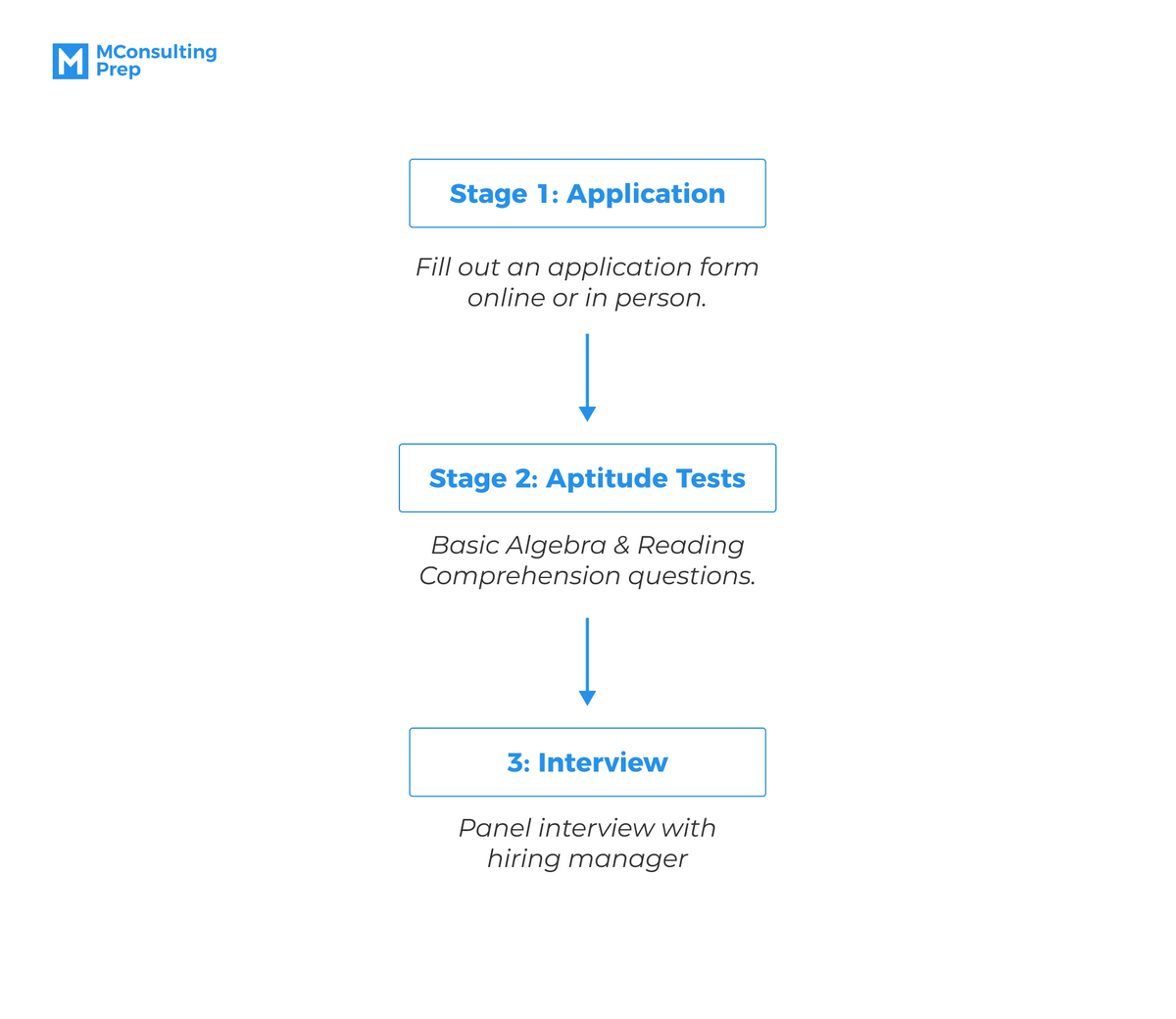Stage 1: Application

Depending on each IBEW local branch, you must fill out an application form online or in person. By that, the company can screen out qualified candidates by looking into their educational background, work history, or skillset. Before applying, all applicants must meet the minimum requirements for an apprenticeship.

Stage 2: Aptitude tests

IBEW aptitude tests consist of basic algebra and reading questions, assessing competencies needed for an electrician: mathematical skills and verbal comprehension. There can also be some mechanical questions, asking you about pulleys, wheels, load distribution, or common mechanical theory, which is the basic foundation of any electrician.

Stage 3: Interview

After passing the aptitude tests, you are invited to a panel interview. This is a comprehensive discussion with the hiring manager to help IBEW better understand your ability, work ethic, and expectations for the role and the company. In this stage, IBEW can determine your rank in the applicant list.

## How to pass IBEW aptitude tests?

Though IBEW uses only 2 types of aptitude tests to filter out candidates: Math Tests and Reading Tests, the assessments are still challenging, especially for the unique format of math questions. The next part of this article will dive deeper into each test type: what to expect and key takeaways to ace these difficult screening tools.

### IBEW Math Tests

In the IBEW Math section, you are required to complete 33 questions about algebra and functions in 46 minutes, by which the company can assess your numerical skills and basic mathematical knowledge. Overall, there are 5 common types of math questions in IBEW Math tests:

• Algebra Equation
• Functions
• Polynomials
• Inequalities
• Number series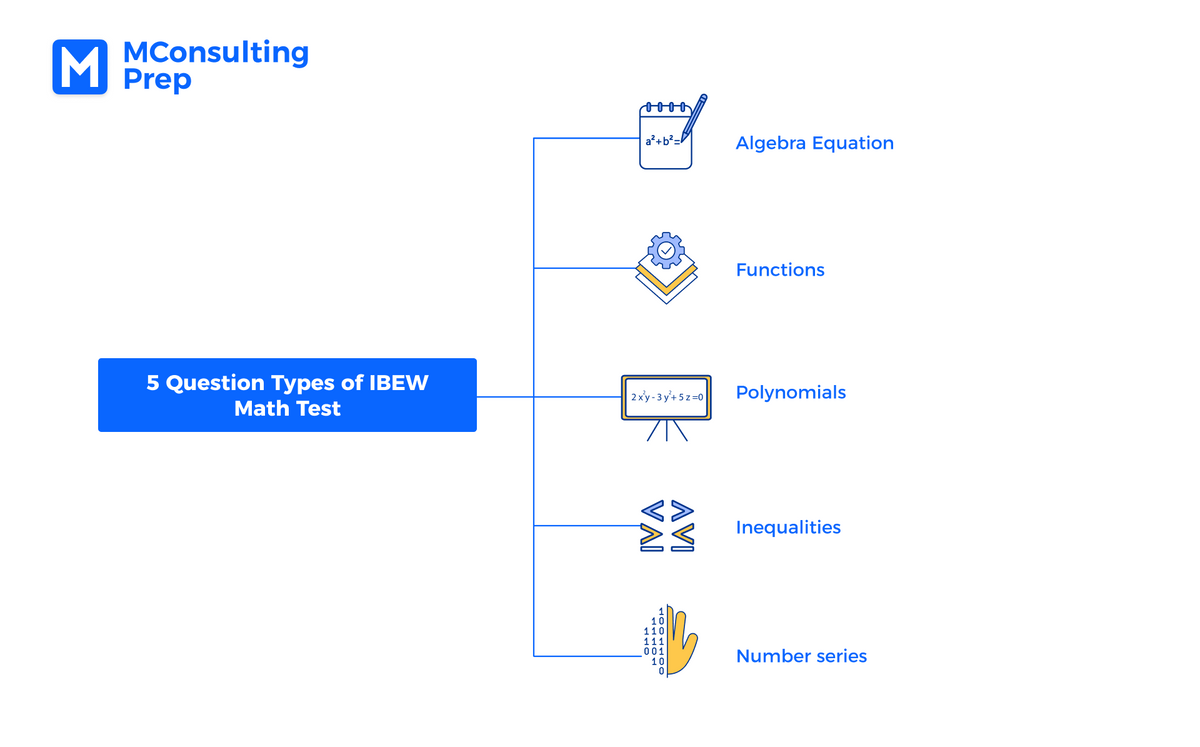Algebra Equation

In this question type, you have to find an unknown factor in a math expression by using basic operations: addition. subtraction, multiplication, or division. This exercise is also known as “finding x”. Here is an example of how the question will be:

Question 1: Find the x

2(3x+1) = 20

Explanation:

2(3x+1) = 20
⇒ 3x+1 = 10
⇒ 3x = 9
⇒ x = 3

KEY TAKEAWAYS

Although this is a fairly straightforward type of math question, it can be super confusing when the expression contains many calculations. The key point is to isolate the factor “x”, which means you should bring the variable to the left side and gather all the remaining values to the right side.

To better illustrate, let’s look at another example:

Question 2: Find the x

4x-5 = 3(x+1) - 4

Explanation:

4x-5 = 3(x+1) - 4
⇒ 4x - 5 = 3x + 3 - 4
⇒ 4x - 3x = 3 - 4 + 5
⇒ x = 4

Functions

This type of problem usually requires you to identify the relationship between x and y based on a given graph. Let’s try a question of this type:

Question 3: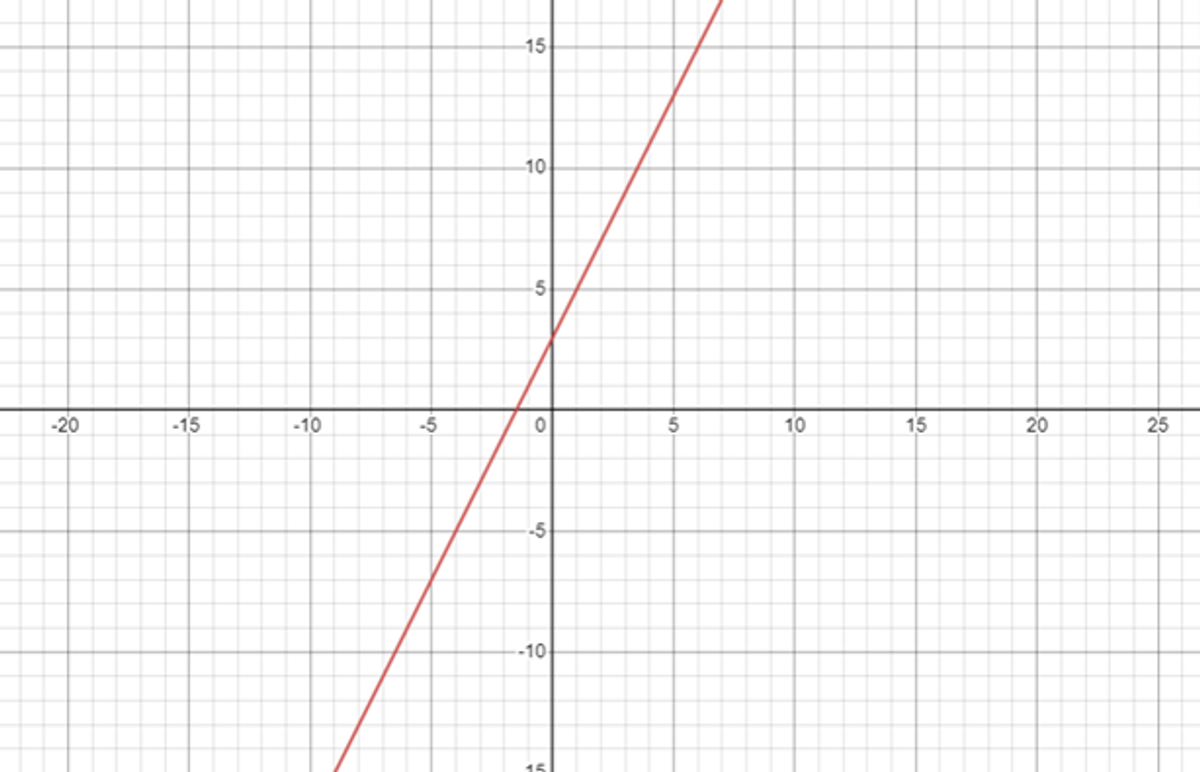Which of the following equations best represents the graph?

A. y = x +4
B. y = 2x + 4
C. y = 2x + 3
D. y = 3x + 2

Explanation:

First, we need to pick any 2 points in the graph.

We have A(0;3) and B(6;15).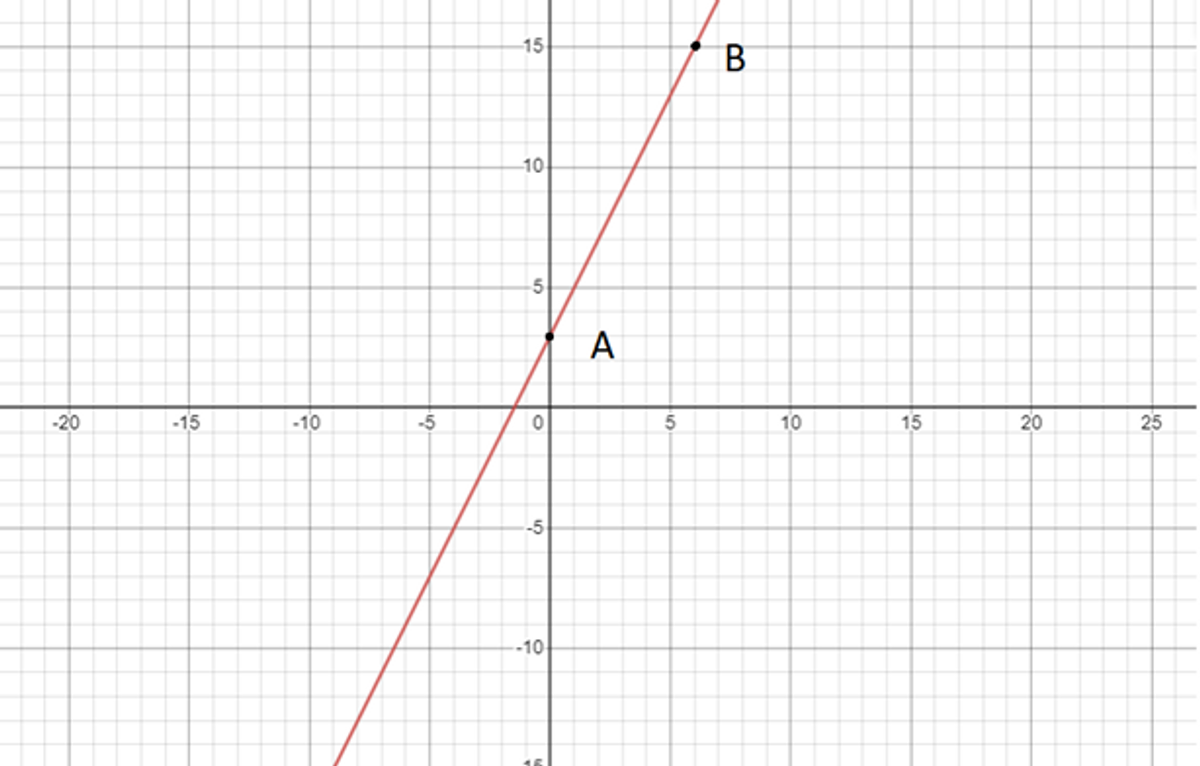Assuming that the function is y = ax + b.

Then, replace x, y with the 2 points you have selected.

We have:

3 = a.0 + b ⇒ b = 3
15 = a.6 + b ⇒ 15 = 6a + 3 ⇒ a = 2

Therefore, the correct answer is y = 2x + 3.

KEY TAKEAWAYS

When picking the 2 points, it is easier to pick x and y as a whole number. Moreover, picking points with a zero coordinate will help you to find a and b in only one step instead of solving a complex equation in 2 variables.

Polynomials

Polynomial questions ask you to simplify or factor polynomials to get a new expression of indeterminates. Let’s look at a sample question to understand how it works:

Question 4: Find the product:

(x - 4)(x2 + 1)

Answer: x3 - 4x2 + x - 4

Explanation:

Multiplying each term in one parenthesis by the terms in the remaining parenthesis, we have:

x.x2 - 4.x2 + x.1 - 4.1
= x3 - 4x2 + x - 4

KEY TAKEAWAYS

To solve polynomial questions, you can follow 3 simple steps:

• Open parentheses
• Combine alike terms
• Rearrange the terms in descending order of exponent

Unlike simplifying questions, factorizing polynomials requires a higher level of math skills as you have to group the common factor among the terms, often in a quadratic equation. Here is an example of a factoring question:

Question 5: Factor the given expression

x2 - 8x + 12

Explanation:

x2 - 2x - 6x + 12
= (x2 - 2x) - (6x - 12)
= x(x-2) - 6(x-2)
= (x-6)(x-2)

Inequalities

Inequality questions include expressions with “>”, “<”, or “≥”, “≤”, which ask you to reason the relationship between 2 required terms. Try out some example questions below:

Question 6: Solve the inequality:

5x -2 ≥ 3x + 10

Explanation:

Bring alike terms to a side.

5x - 3x ≥ 10 + 2
⇒ 2x ≥ 12
⇒ x ≥ 6

NOTE

Unlike equations, you need to keep in mind these guidelines when dealing with this question type:

• Don't multiply or divide by a variable (for example, multiplying 2 sides by x)
• Change the direction of the inequality if you multiply or divide by a negative number (for example, multiplying 2 sides by -2)

Let’s apply these tips into another example:

Question 7: Solve the inequality:

-5 - 2x ≥ -7 + x

Explanation:

Multiply 2 sides by -1

⇒ 5 + 2x ≤ 7 - x
⇒ 5-7 ≤ -x -2x
⇒ -2 ≤ -3x
⇒ 2 ≥ 3x
⇒ x ≤ 2/3

Number Series

In this question, you are presented with an arithmetic sequence with one missing number, which requires you to determine by finding the pattern it follows.

Question 8: Find the question mark

12, 15, ?, 21, 24, 27

Explanation:

Each number of the sequence is 3 more than the previous number:

15=12+3
24=21+3
27=24+3

So the answer will be: 15+3 = 18.

NOTE

To solve this test type, you need to find the relation between each pair of consecutive numbers by using basic math calculations: addition, subtraction, multiplication, or division. Once noticing the shared pattern in all pairs, you can generate the missing term by making the same calculation to its predecessor or successor.

Let’s practice another question:

Question 9: Find the missing number:

2, 8, 20, ?, 92, 188

Explanation:

Each number in the sequence is generated by adding 2 to the previous term, then multiplying by 2.

8 = (2+2)x2
20 = (8+2)x2
188 = (92+2)x2

Therefore, the correct answer is: (20+2)x2 = 44

Reading Comprehension in IBEW aptitude tests asks you to find a correct answer after reading a passage followed by some questions. The whole test contains 36 questions under a time limit of 51 minutes. IBEW Reading Tests aim to measure your ability to process written data and make inferences from that. Here is an official sample question from IBEW:

Question 1:

The timing of New Year’s Day has changed with customs and calendars. The Mayan civilization, on what is now called the Yucatan peninsula of Mexico, celebrated the New Year on one of the two days when the noonday sun is directly overhead. In the equatorial regions of the earth, between the Tropics of Cancer and Capricorn, the sun is in this position twice a year, once on its passage southward, and once on its passage northward. At the early Mayan city of Izapa in the southern Yucatan, the overhead date for the sun on its southward passage was August 13. The Mayans celebrated this as the date for the beginning of the New Year. Later at the more northerly Mayan site at Edzna, the corresponding overhead date is July 26. Analyses of Mayan pictorial calendars indicate that they celebrated the New Year on August 13 prior to 150 AD, and on July 26 after that year. This change has been explained by archaeological dating showing that 150 AD was the time that the Mayans moved the hub of their civilization from the southern to the northern site.

If the Mayans had moved their civilization’s center south of Izapa, their new date for the celebration of the New Year would probably have been closest to which of the following dates?

A. January 1
B. February 20
C. March 25
D. September 15

Explanation:

The passage states “At the early Mayan city of Izapa in the southern Yucatan, the overhead date for the sun on its southward passage was August 13.” Since the Mayans “celebrated the New Year on one of the two days when the noonday sun is directly overhead.” ⇒ September 15 is closest to August 13.

Therefore, the correct answer is D.

1. How can I prepare for the IBEW aptitude tests?

One proven tip is to practice as much as possible. Fortunately, there are official questions from IBEW local websites to help you have a good grasp of the format, especially the challenging math section. You can also enhance your skills by practicing numerical reasoning and verbal reasoning mock tests from prestige test providers like SHL, Aon, or Saville.

2. Can I retake the IBEW aptitude tests?

If you failed the IBEW aptitude tests, don’t be afraid as you can reapply and retake the tests after 6 months. However, there will be a record of your previous attempt, so try to prepare carefully for the selective recruitment at IBEW.

3. What can I take to with me to the IBEW aptitude tests?

A Photo ID is required. As IBEW states, “No ID, no test-no exceptions!”
For the math section, you are not allowed to use a calculator. Pencils and scrap paper are provided for computation. Therefore, you don’t need to prepare anything except for the vital Photo ID.

4. How can I know if I passed the IBEW aptitude tests?

After about 2-4 weeks, there will be an email announcing that you’ve passed the tests, and information about the date and location for an in-person interview. However, the company will not provide you with a review of your performance. The only thing you will be received is whether you passed or failed.

Related product